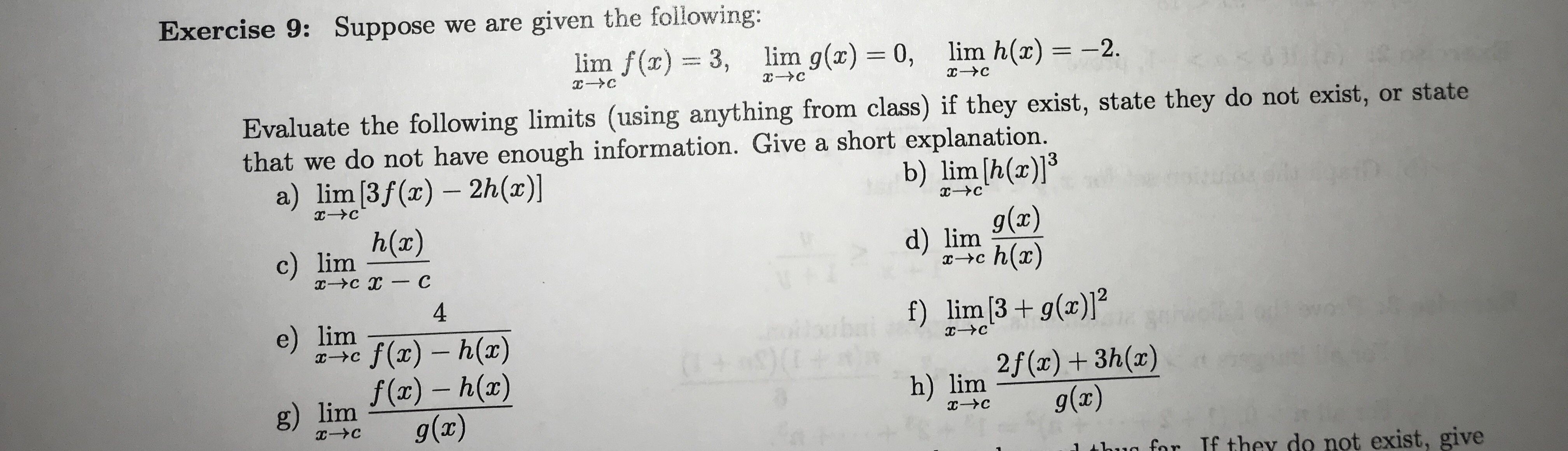# Exercise 9: Suppose we are given the following:lim f(x)3,lim g(x) 0, lim h(x) -2CCEvaluate the following limits (using anything from class) if they exist, state they do not exist, or statethat we do not have enough information. Give a short explanation.a) lim [3f (x) 2h(x)]b) lim [h(a)]h(x)g(x)d) limh(ar)c) lim-Ус х — С4f) lim [3g(x)]e) limilbuba(+ 9 0f(x) h(a)f(ar)-h(ar)g(x)CIXC2f(x)3h(a)g(x)IXh) limg) limс-Усx-Cfor If they do not exist, give

Question
8 viewshelp_outlineImage TranscriptioncloseExercise 9: Suppose we are given the following: lim f(x)3, lim g(x) 0, lim h(x) -2 C C Evaluate the following limits (using anything from class) if they exist, state they do not exist, or state that we do not have enough information. Give a short explanation. a) lim [3f (x) 2h(x)] b) lim [h(a)] h(x) g(x) d) lim h(ar) c) lim -Ус х — С 4 f) lim [3g(x)] e) lim ilbuba (+ 9 0 f(x) h(a) f(ar)-h(ar) g(x) C IXC 2f(x)3h(a) g(x) IX h) lim g) lim с-Ус x-C for If they do not exist, give fullscreen
check_circle

Step 1

Hey, since there are multiple subparts posted, we will answer first 3 questions. If you want any specific question to be answered then please submit that question only or specify the question number in your message

Step 2

(a)

Find the limit of the function as x approaches c as follows.

Step 3

b)

Find the limit of the function as x appro...

### Want to see the full answer?

See Solution

#### Want to see this answer and more?

Solutions are written by subject experts who are available 24/7. Questions are typically answered within 1 hour.*

See Solution
*Response times may vary by subject and question.
Tagged in

### Math